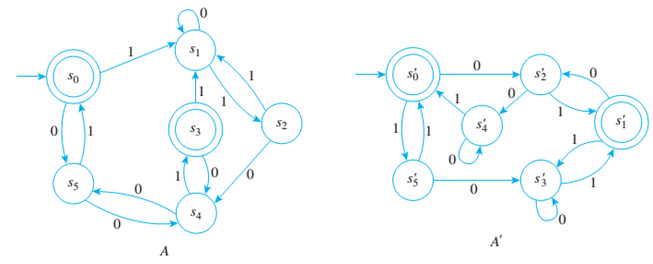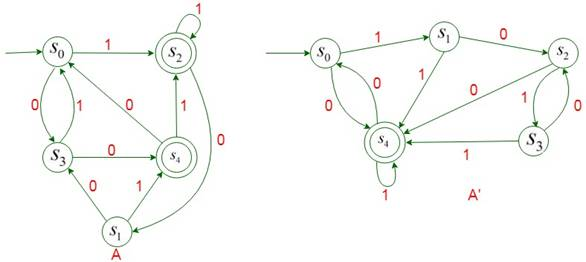Chapter 12.3, Problem 9ES### Discrete Mathematics With Applicat...

5th Edition
EPP + 1 other
ISBN: 9781337694193

#### Solutions

Chapter
Section### Discrete Mathematics With Applicat...

5th Edition
EPP + 1 other
ISBN: 9781337694193
Textbook Problem
1 views

# Are the automata A and A’ shown below equivalent?To determine

Are automata A,A' equivalent.Explanation

Given information:

Concept used:

If the quotient transition diagram of the two automata are same then the two automata are equivalent.

Calculation:

For A :

0 Equivalence classes - Two states are zero equivalent if both are accepting states or both are non-accepting states. Therefore, the 0-equivalance classes are: {s1,s2,s4,s5},{s0,s3}.

1 Equivalence classes — Two states are 1 equivalent if, and only if they are 0 equivalent and, after input of any symbol, their next state are 0 equivalent. Therefore, the 1 equivalence classes are: {s1,s2},{s4,s5},{s0,s3}

2 Equivalence classes — two states are called 2 equivalent if, 2 and only ¡f they are 1 equivalent and. after input of any symbol, their next state are 1 equivalent. Therefore the 2 equivalent classes are: {s1},{s2},{s4,s5},{s0,s3}

3 Equivalence classes — two states are called 3 equivalent if, and only if they are 2 equivalent and. after input of any symbol, their next state are 2 equivalent

### Still sussing out bartleby?

Check out a sample textbook solution.

See a sample solution

#### The Solution to Your Study Problems

Bartleby provides explanations to thousands of textbook problems written by our experts, many with advanced degrees!

Get Started